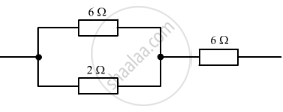Share

# The Figure Given Below Shows Three Resistors? - CBSE Class 10 - Science

ConceptResistance of a System of Resistors - Resistors in Parallel

#### Question

The figure given below shows three resistors?Their combined resistance is:

(a) 1 5/7Ω

(b) 14 Ω

(c) 6 2/3 Ω

(d) 7 1/2 Ω

#### Solution

(d)   7 1/2Ω

The resistors of 6and 2 are connected in parallel

∴  1/R=1/R_1+1/R_2

Here,

R_1=6Ω

R_2=2 Ω

1/R=1/6+1/2

1/R=4/6

R=6/4

This arrangement is further connected in series with the 6 resistor

∴Net resistance =6/4+6=7 1/2 Ω

Is there an error in this question or solution?

#### Video TutorialsVIEW ALL 

Solution The Figure Given Below Shows Three Resistors? Concept: Resistance of a System of Resistors - Resistors in Parallel.
S